# Steffen, Eckhard

## Circular flow numbers of $r$-graphs ★★

Author(s): Steffen

A nowhere-zero-flowonis an orientation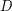oftogether with a functionfrom the edge set ofinto the real numbers such that, for all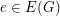, and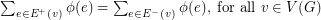.

A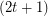-regular graphis a-graph if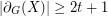for everywith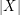odd.

Conjecture   Let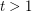be an integer. Ifis a-graph, then.

Keywords: flow conjectures; nowhere-zero flows

## Circular flow number of regular class 1 graphs ★★

Author(s): Steffen

A nowhere-zero-flowonis an orientationoftogether with a functionfrom the edge set ofinto the real numbers such that, for all, and. The circular flow number ofis inf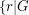has a nowhere-zero-flow, and it is denoted by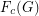.

A graph with maximum vertex degreeis a class 1 graph if its edge chromatic number is.

Conjecture   Letbe an integer anda-regular graph. Ifis a class 1 graph, then.